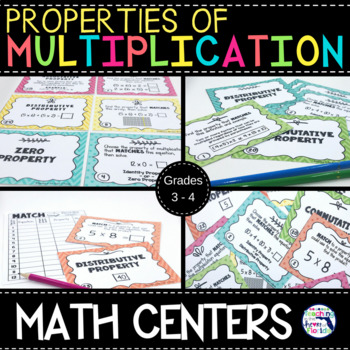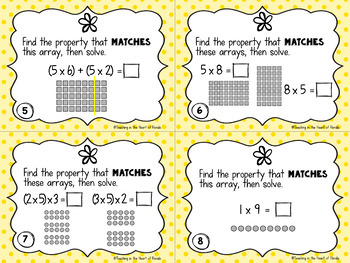# Properties of Multiplication Activities Math Centers

Rated 4.8 out of 5, based on 5 reviews
5 Ratings;
2nd - 4th, Homeschool
Subjects
Resource Type
Standards
Formats Included
• Zip
Pages
37 pages
Report this resource to TPT

### Description

Mastering multiplication properties requires practice and these matching task cards will give your 3rd and 4th graders and homeschool students meaningful and rigorous practice with five different multiplication properties matching activities! They give students many different ways to work on procedural, conceptual, and application levels of understanding with the Commutative, Associative, Distributive, Identity, and Zero Properties of Multiplication. Flexible, versatile, and perfect for differentiation – this resource can be used as games, centers, small group intervention, early and fast finishers and much more!

PLEASE SEE PREVIEW FOR A CLOSER LOOK!

Included in this resource:

♦ 5 sets – 24 task cards each:

1. ”Property” Matchdefinitions and examples help students initially identify the different properties.

2. “Equation to Property” Match Students are given two arrays with the same product which they will match to the two matching equations.

3. “Arrays, Equations, Property” Match - Students are given multiplication equations & arrays to help them "see" how the properties help them multiply.

4. “Choose the Property ” Match - Students are given a choice of one of two properties that will match the multiplication equation.

5. “Select a Property” Match - Students are multiplication equations and given the opportunity to select the property that they can apply to solve the equation.

Centers 2, 3, 4, and 5 include the opportunity for students to solve the multiplication equations for extra rigor!

♦ 5 Answer Keys for easy self- checking

♦ 1 Student Recording Sheet

This resource is perfect for:

• Math Centers

• Concentration Games

• Partner Quizzing

• Independent Practice

• Small Group Intervention

• Early and Fast Finishers

More Math Resources in My Store:

♦ ♦ ♦ ♦ ♦ ♦ ♦ ♦ ♦ ♦ ♦ ♦ ♦ ♦ ♦ ♦ ♦ ♦ ♦ ♦ ♦ ♦ ♦ ♦ ♦ ♦ ♦ ♦ ♦ ♦ ♦ ♦ ♦ ♦ ♦ ♦ ♦ ♦ ♦ ♦ ♦ ♦ ♦ ♦ ♦ ♦ ♦ ♦ ♦ ♦ ♦ ♦ ♦ ♦ ♦ ♦ ♦ ♦ ♦ ♦ ♦ ♦ ♦ ♦ ♦ ♦ ♦

♦ ♦ ♦ ♦ ♦ ♦ ♦ ♦ ♦ ♦ ♦ ♦ ♦ ♦ ♦ ♦ ♦ ♦ ♦ ♦ ♦ ♦ ♦ ♦ ♦ ♦ ♦ ♦ ♦ ♦ ♦ ♦ ♦ ♦ ♦ ♦ ♦ ♦ ♦ ♦ ♦ ♦ ♦ ♦ ♦ ♦ ♦ ♦ ♦ ♦ ♦ ♦ ♦ ♦ ♦ ♦ ♦ ♦ ♦ ♦ ♦ ♦ ♦ ♦ ♦ ♦

"Don't forget to leave feedback -you will receive TPT credits that you can use toward future purchases!

Thank you for visiting Teaching in the Heart of Florida!

Total Pages
37 pages
Included
Teaching Duration
N/A
Report this resource to TPT
Reported resources will be reviewed by our team. Report this resource to let us know if this resource violates TPT’s content guidelines.

### Standards

to see state-specific standards (only available in the US).
Interpret products of whole numbers, e.g., interpret 5 × 7 as the total number of objects in 5 groups of 7 objects each. For example, describe a context in which a total number of objects can be expressed as 5 × 7.
Apply properties of operations as strategies to multiply and divide. Examples: If 6 × 4 = 24 is known, then 4 × 6 = 24 is also known. (Commutative property of multiplication.) 3 × 5 × 2 can be found by 3 × 5 = 15, then 15 × 2 = 30, or by 5 × 2 = 10, then 3 × 10 = 30. (Associative property of multiplication.) Knowing that 8 × 5 = 40 and 8 × 2 = 16, one can find 8 × 7 as 8 × (5 + 2) = (8 × 5) + (8 × 2) = 40 + 16 = 56. (Distributive property.)